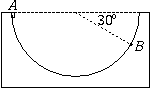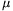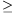Középiskolai Matematikai és Fizikai Lapok
Informatika rovattal
 Már regisztráltál? Új vendég vagy?# Exercises and problems in Physics January 1999

## New experimental problem:

m. 203. Measure the densities of lump sugar, granulated sugar, icing sugar and Christmas fondant as accurately as possible. How does the water content affect the densities?

## New exercises:

FGy. 3215. How much energy is necessary to tear a CO2 molecule from solid carbon-dioxide? (The required data can be found in scientific tables.)

FGy. 3216. A caterpillar tractor moves on a horizontal road at a speed of 36 km/h. a) Determine the kinetic energy of one of the 600 kg caterpillars. b) What is the kinetic energy of this caterpillar according to the driver of the tractor?

FGy. 3217. People at an excursion measure the boiling point of water to be 99 oC at the morning start and 97 oC at sunset, at the end of a walk in a hilly country. Using scientific tables, estimate the difference in the altitudes of the two places. The temperature is 15 oC at both places and times, and the atmospheric pressure does not change during the day.

## New problems:

FF. 3218. The period of a binary system is 2 years, the distance between the two stars is 300 million kilometres and the mass of one of the stars equals that of the Sun. What is the mass of the other star?FF. 3219. A small, flat body is released with no initial speed and slides down from the rim (point A in the figure) of a fixed, half-cylinder shaped trough, the axis of which is horizontal. a) Does the body slide to point B if the coefficient of friction between the body and the trough is1=0.5? b) Does the body reach point B if the coefficient of friction is2=0.268?

FF. 3220. A point-like body with a mass of 0.5 kg moving at a uniform speed of 5 m/s on an elliptical track experiences a force of 10 N at the endpoints of the major axis and a force of 1.25 N at the endpoints of the minor axis. How long are the axes of the ellipse?

FF. 3221. When is the earliest time after midnight when the distance between the endpoints of the hands of the clock in exercise 3214 changes the most rapidly? And the most slowly?

FF. 3222. A light with a wavelength of 0.4m and an intensity of 0.5 W/cm2 falls perpendicularly onto the surface of a cesium photo-cathode. The exit energy of the electrons is 2 eV. If the electrons leave the surface perpendicularly, what pressure do they exert on it? How does this relate to the pressure of the incident light? (Assume that half of the incident photons is absorbed, the rest is reflected perpendicularly, and 10 percent of the absorbed photons produce photoelectrons.)

FN. 3223. A homogeneous rod of mass m and length l is held horizontally by supporting its two ends with our fingers. When we slowly approach our fingers together, they finally meet under the centre of the rod, while the rod slips on either one or the other finger. How much work do we do during the process if the coefficient of static friction iss, that of dynamic friction isd (sd)?